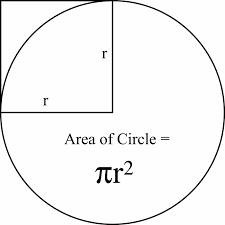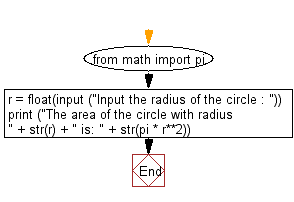﻿ Python: Input the radius of a circle and compute the area - w3resource# Python: Input the radius of a circle and compute the area

## Python Basic: Exercise-4 with Solution

Write a Python program which accepts the radius of a circle from the user and compute the area.

Python: Area of a Circle

In geometry, the area enclosed by a circle of radius r is πr2. Here the Greek letter π represents a constant, approximately equal to 3.14159, which is equal to the ratio of the circumference of any circle to its diameter.Sample Solution:-

Python Code:

``````from math import pi
r = float(input ("Input the radius of the circle : "))
print ("The area of the circle with radius " + str(r) + " is: " + str(pi * r**2))
```
```

Sample Output:

```Input the radius of the circle : 1.1
The area of the circle with radius 1.1 is: 3.8013271108436504
```

Flowchart:## Visualize Python code execution:

The following tool visualize what the computer is doing step-by-step as it executes the said program:

Python Code Editor:

Have another way to solve this solution? Contribute your code (and comments) through Disqus.

What is the difficulty level of this exercise?

Test your Python skills with w3resource's quiz

﻿

## Python: Tips of the Day

Try-catch-else construct:

```try:
foo()
except Exception:
print("Exception occured")
else:
print("Exception didnt occur")
finally:
print("Always gets here")
```